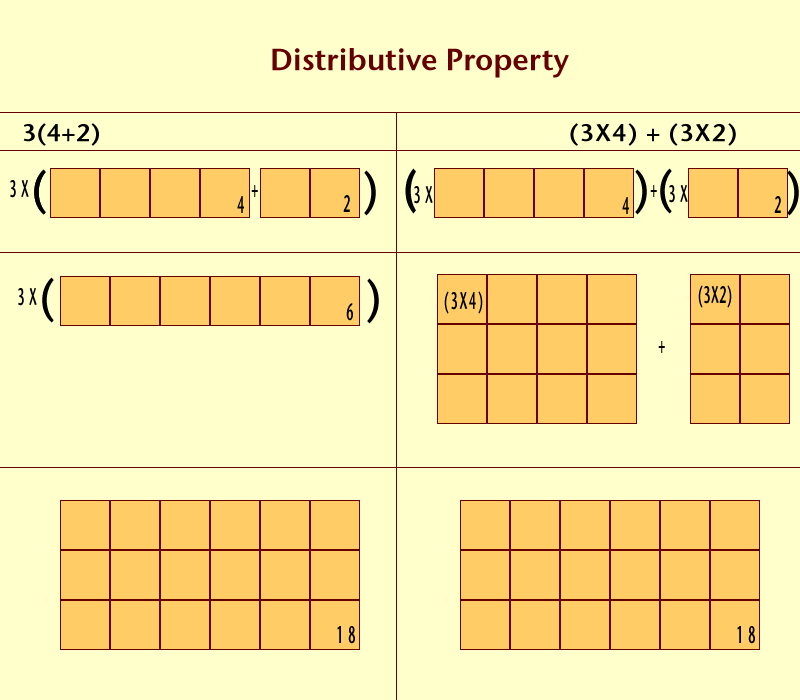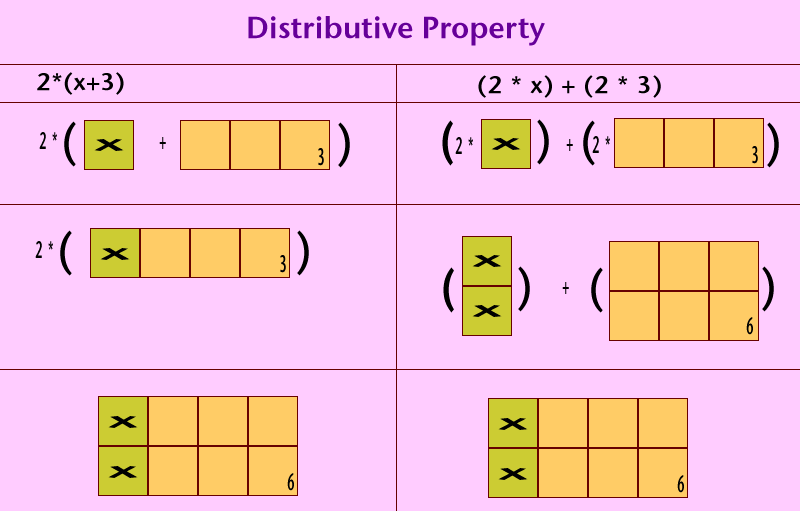Distributive Property -- Created for Pre-Algebra -- by: Alicia Klaassen

Name:_____________________________________________ Date:_________

Example: 3(4 + 2)Use pictures of rectangles and their areas to model the distributive property for each numerical expression.

1. 2(6 + 4)                                                                 2. 4(5 + 8)                              3. (8 + 3)5                                                                  4. (6 + 9)2

Example: 2(x + 3)Use the pictures of algebra tiles and their areas to model the distributive property for each algebraic expression.

1. 3( x + 1)                                                                 2. 2(x + 4)

3. (y + 4)5                                                                  4. (y + 3) 6

5. 4(x – 2)                                                                   6. 2(n + 4)